# Fraction calculator

The calculator performs basic and advanced operations with fractions, expressions with fractions combined with integers, decimals, and mixed numbers. It also shows detailed step-by-step information about the fraction calculation procedure. Solve problems with two, three, or more fractions and numbers in one expression.

## Result:

### (31/5) : 4 = 4/5 = 0.8

Spelled result in words is four fifths.

### How do you solve fractions step by step?

1. Conversion a mixed number 3 1/5 to a improper fraction: 3 1/5 = 3 1/5 = 3 · 5 + 1/5 = 15 + 1/5 = 16/5

To find a new numerator:
a) Multiply the whole number 3 by the denominator 5. Whole number 3 equally 3 * 5/5 = 15/5
b) Add the answer from previous step 15 to the numerator 1. New numerator is 15 + 1 = 16
c) Write a previous answer (new numerator 16) over the denominator 5.

Three and one fifth is sixteen fifths
2. Divide: 16/5 : 4 = 16/5 · 1/4 = 16 · 1/5 · 4 = 16/20 = 4 · 4 /4 · 5 = 4/5
Dividing two fractions is the same as multiplying the first fraction by the reciprocal value of the second fraction. The first sub-step is to find the reciprocal (reverse the numerator and denominator, reciprocal of 4/1 is 1/4) of the second fraction. Next, multiply the two numerators. Then, multiply the two denominators. In the following intermediate step, cancel by a common factor of 4 gives 4/5.
In other words - sixteen fifths divided by four = four fifths.

#### Rules for expressions with fractions:

Fractions - simply use a forward slash between the numerator and denominator, i.e., for five-hundredths, enter 5/100. If you are using mixed numbers, be sure to leave a single space between the whole and fraction part.
The slash separates the numerator (number above a fraction line) and denominator (number below).

Mixed numerals (mixed fractions or mixed numbers) write as integer separated by one space and fraction i.e., 1 2/3 (having the same sign). An example of a negative mixed fraction: -5 1/2.
Because slash is both signs for fraction line and division, we recommended use colon (:) as the operator of division fractions i.e., 1/2 : 3.

Decimals (decimal numbers) enter with a decimal point . and they are automatically converted to fractions - i.e. 1.45.

The colon : and slash / is the symbol of division. Can be used to divide mixed numbers 1 2/3 : 4 3/8 or can be used for write complex fractions i.e. 1/2 : 1/3.
An asterisk * or × is the symbol for multiplication.
Plus + is addition, minus sign - is subtraction and ()[] is mathematical parentheses.
The exponentiation/power symbol is ^ - for example: (7/8-4/5)^2 = (7/8-4/5)2

#### Examples:

subtracting fractions: 2/3 - 1/2
multiplying fractions: 7/8 * 3/9
dividing Fractions: 1/2 : 3/4
exponentiation of fraction: 3/5^3
fractional exponents: 16 ^ 1/2
adding fractions and mixed numbers: 8/5 + 6 2/7
dividing integer and fraction: 5 ÷ 1/2
complex fractions: 5/8 : 2 2/3
decimal to fraction: 0.625
Fraction to Decimal: 1/4
Fraction to Percent: 1/8 %
comparing fractions: 1/4 2/3
multiplying a fraction by a whole number: 6 * 3/4
square root of a fraction: sqrt(1/16)
reducing or simplifying the fraction (simplification) - dividing the numerator and denominator of a fraction by the same non-zero number - equivalent fraction: 4/22
expression with brackets: 1/3 * (1/2 - 3 3/8)
compound fraction: 3/4 of 5/7
fractions multiple: 2/3 of 3/5
divide to find the quotient: 3/5 ÷ 2/3

The calculator follows well-known rules for order of operations. The most common mnemonics for remembering this order of operations are:
PEMDAS - Parentheses, Exponents, Multiplication, Division, Addition, Subtraction.
BEDMAS - Brackets, Exponents, Division, Multiplication, Addition, Subtraction
BODMAS - Brackets, Of or Order, Division, Multiplication, Addition, Subtraction.
GEMDAS - Grouping Symbols - brackets (){}, Exponents, Multiplication, Division, Addition, Subtraction.
Be careful, always do multiplication and division before addition and subtraction. Some operators (+ and -) and (* and /) has the same priority and then must evaluate from left to right.

## Fractions in word problems:

• Third of an hour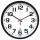How many minutes is a third of an hour? Do you know to determine a third of the lesson hour (45min)?
• Division by zero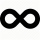Fraction 5 by 2. if 3 is added to numerator and 2 is subtracted from the denominator then the new fraction is:
• Joel divided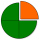Joel divided a number and got a solution of 8 quarters(1/4). What is the whole number that can be divided into 8 quarters
• Kate shares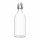Kate shares a 64-ounce bottle of apple cider with five friends. Each person's serving will be the same number of ounces. Between what two whole number of ounces will each person's serving to be? Explain using division.
• Max has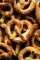Max has a 1/3 of a pound of pretzels and wants to share equal parts between himself and a friend. How much pretzels will Max's friend receive?
• Dividing walnuts into crates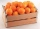There are 8 and 2 over 3 pounds of walnuts in a container, which will be divided equally into containers that hold 1 and 1 over 5 pounds. This would fill n and 4 over 18 containers. What is n?
• Statues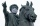Diana is painting statues. She has 7/8 of a liter of paint remaining. Each statue requires 1/20 of a liter of paint. How many statues can she paint?
• A serving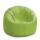A serving of rice is 1/2 of a cup. How many servings are there in a 3 1/2-cup bag of rice?
• Mrs. Zarka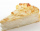Mrs. Zarka has 3 pies for a party. She calculates that if she splits the pies evenly among the guests, they will each receive 1/6 of a pie. How many guests are there?
• Nicola 2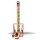Nicola is playing a video game where each round lasts 7/12 of an hour. She has scheduled 3 3/4 hours to play the game. How many rounds can Nicole play?
• Equivalent expressionsA coach took his team out for pizza after their last game. There were 14 players, so they had to sit in smaller groups at different tables. Six players sat at one table and got 4 small pizzas to share equally. The other players sat at the different table
• Nicolas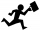Nicolas and his father can repair one desk in 1/3 hour. How many desks can they repair in 3 hours?
• Janna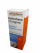Janna takes her medicine 3 times a day. How many days will a 60 ml medicine last if 2 1/2ml is taken each time?